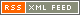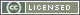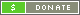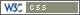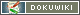# Noise Figure Calculations

Loren Moline WA7SKT wrote:

Is it possible to calculate the improved signal to noise ratio between a 1.5db NF LNA and a .6db NF LNA?

Loren WA7SKT

Loren

wonderful design tool. One of the features is a “signals and Systems”
section that allows you to do exactly what you are talking about.
Every segment of a system is entered with its associated loss or gain
and noise figure, where appropriate. Then the complete system noise
figure, gain and S/N is calculated. Any component can then be altered
to see what effect that alteration will have on the system performance.

It might be a bit difficult to find where that download is on the
website. It is a very busy website.

73,

Dave, K4TO

Loren,

I'm not so sure that AapCad computes with a non room temp antenna target….

Well, for you to understand it before using software, here it goes.

The NF is a measure of the degradation of the signal to noise in systems referenced to room temperature.

If all is at 290K then you have 6dB s/n with a 1.5dB NF system and you will have 7dB s/n with a 0.5dB NF system

Rapid calculations with NF can be done in cases like this with simple addition and subtraction:

If you are pointing antennas to the sky and have non 290K target then the story is completely different. You need to calculate it all. Best is to work with noise temperature equivalents than all adds up quite nicely.

NF= 10*log(T/290 + 1)

1. your system NF of 1.5dB equals 120K noise temp.
2. your system NF of 0.5dB equals 35K noise temp.

So if you're pointing to the sky to a spot with about 30K of equivalent noise temperature, your system in 1) sees a total noise 120+30=150K your system in 2) sees a total noise 35+30=65K, so your system in 2) is 150/65 times quieter, that is 2.3 times better, that is it has a 3.6dB better S+N/N ratio.

If you had a 6dB s/n in 1) you will have 9.6dB s/n in 2)

So when pointing to lower temp places the improvement in s/n is bigger than the reduction in the NF.

So it is possible to calculate providing you know what is the noise temp. the antenna is pointing to.

Luis Cupido, ct1dmk

P.S. now calculate if you're at microwaves and point to a 5K spot in the sky…
P.P.S. note that I wrote system NF and not preamplifier NF !!! You must compute the total system NF where your preamp is, must include second stages feed losses etc…

Ed Cole Edward Cole kl7uw added:

My EME pathlink calculator does this in the first section: It calculates cascade NF and NT for a three stage receiver including feedlines and antenna temp.

Then it enters the sky temp the antenna is looking at to produce the standard Pn=KTB noise power number.

By comparing Pn (0.5dBNF) to Pn (1.5dBNF) you will have the improvement factor in dB you are seeking. There are three columns so you can compare three examples side by side (I have sample numbers entered).

The program also calculates pathloss to the Moon and S/N, but you can use the first section alone for receiver noise performance.